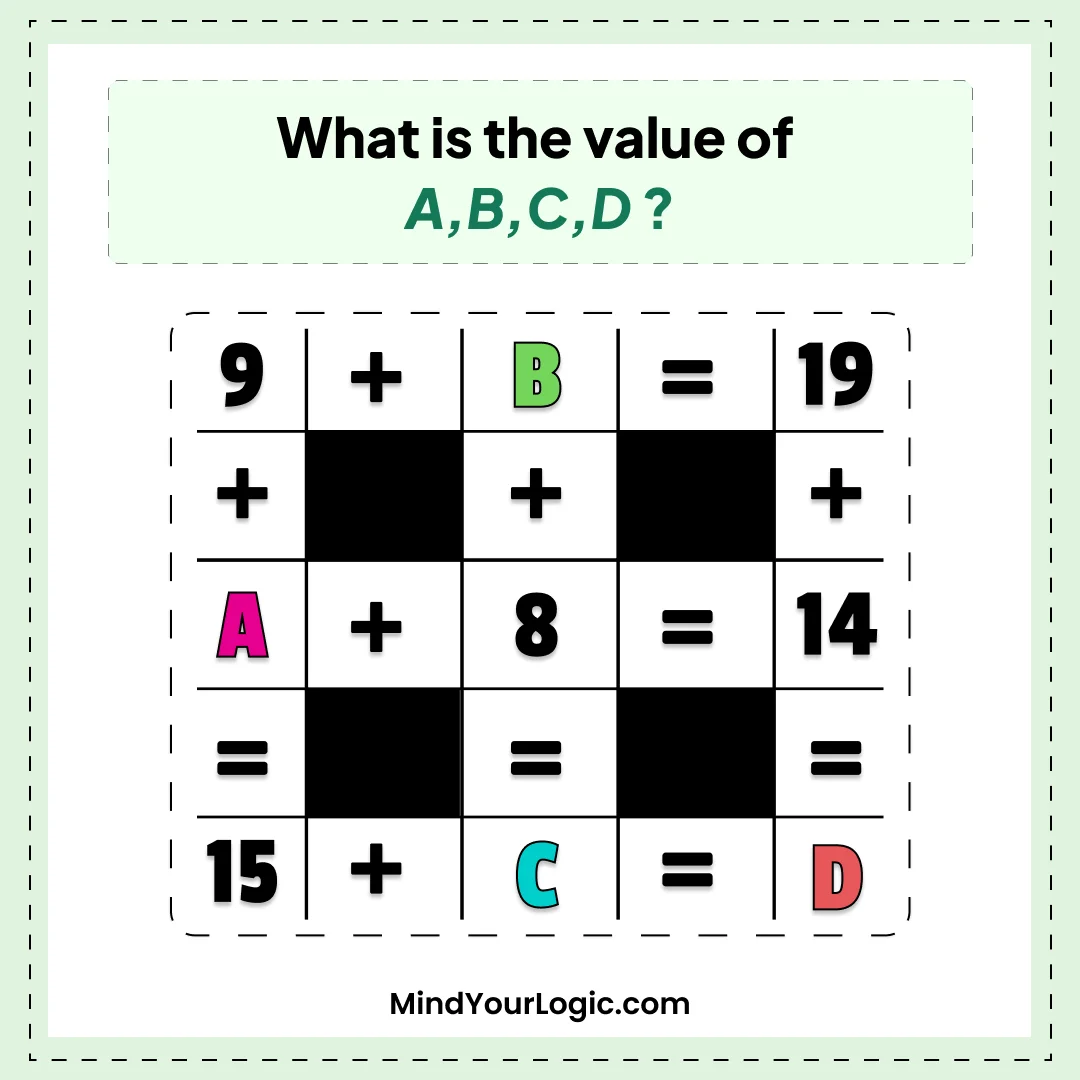# value of ABCD - Math Riddle

###### 87.Math Riddles
`What is the value of A,B,C,D?`• ```
A+8=14
A= 6
9+B = 19
B = 6
D = 19+14 =33
15+ C = D
C = 33-15 = 18```
```Explanation :

A = 6. B = 10. C = 18. D = 33```